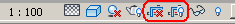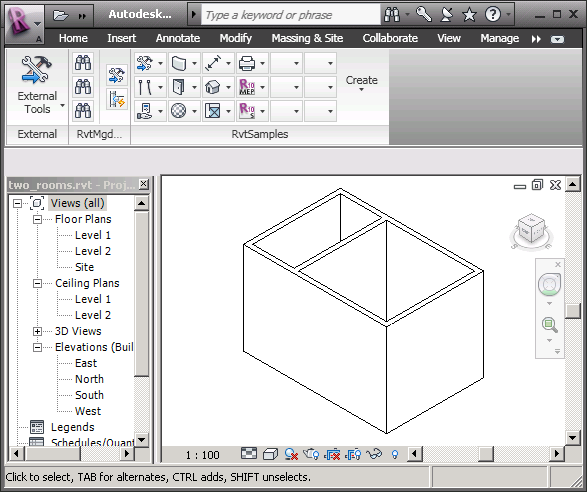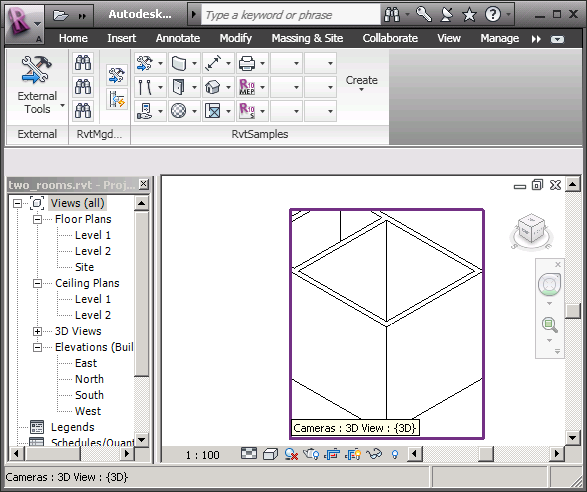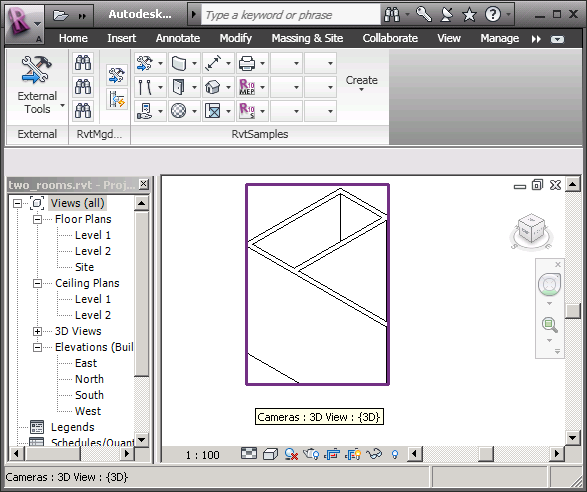### Crop 3D View to Room

In case you have heard enough about both climbing and AU and AUv in the last few posts and would prefer to read about the Revit API and nothing but the Revit API, here is something for you. This is based on a recent case handled by Joe Ye and deals with the 3D view crop box and the transformations required to set it up properly, an issue we have never previously looked at.

Question: I would like to set the crop box of a 3D view to correspond to a single room.

Answer: As the 3D view rotates the room, the original bounding box of the plan view will not be suitable to use for the 3D view crop box. Here is an idea to calculate the crop box. Retrieve all the corner point vertex coordinates of the room, and project them onto the plane perpendicular to the 3D view's view direction. This produces projected point coordinates. From these result point coordinates, determine the maximum and minimum X and Y values. From the max and min points, create the 3D view's crop box.

Here are the detailed steps to implement this idea:

• Retrieve all vertex coordinates of the room.
• Project these coordinates to the plane perpendicular to the 3D view's view direction. To do so, create a face perpendicular to the view direction. The projected results can be obtained from the Face.Project method taking an XYZ point argument. For more information, see the Revit API help file RevitAPI.chm for the usage and return value of this method.
• Go through the resulting coordinates and determine the maximum and minimum X and Y coordinate values.
• Use the four values to determine a bounding box:
```BoundingBoxXYZ bb = new BoundingBoxXYZ();
bb.Min = NewXYZ( xMin, yMin, 0 );
bb.Max = NewXYZ( xMax, yMax, 0 );
```
• Use the bounding box to set the 3D view crop box:
```View3d.CropBox = bb;
```

Actually, after some further investigation, I found a better way to determine the room extents. It is hard to create a solid and make one face of it perpendicular to the view direction.

You can also use the Transform class to calculate the crop box of the 3D view instead.

First, determine the transformation for translating coordinates in the 3D view to the world coordinate system WCS from the View3d.CropBox.Transform property. Then invert it to obtain the transformation to translate model WCS coordinates back to the 3D view coordinates.

Determine the room vertices from its ClosedShell property, and translate them to 3D view coordinates. Find the minimum and maximum X and Y coordinate values as described above and set the 3D view's crop box Max and Min properties using these.

You do need to activate and make the crop box visible to see the effect. You can do so using the Crop View and Show Crop Region buttons in the user interface:This can also be achieved programmatically using:

```  view3d.CropBoxActive = true;
view3d.CropBoxVisible = true;
```

So far for Joe's idea. Many thanks to Joe for all the research into this!

I implemented a new Building Coder sample command CmdCropToRoom to test and demonstrate this. In order to show how the crop box can be set to various different regions in the model, it iterates over all the rooms in the model and sets the crop box of the current 3D view to the next room in the sequence on each call. We retrieve a list of all rooms in the model using doc.get_Elements:

```List<RvtElement> rooms = new List<RvtElement>();
int n = doc.get_Elements( typeof( Room ), rooms );
```

To loop through the rooms in the model and step to the next room in the list on each invocation of the command, we use their index in the list of elements returned by this call. The last index used is stored in a static variable _i, and the method BumpRoomIndex is used to increment it by one on each call:

```static int _i = -1;

static int BumpRoomIndex( int room_count )
{
++_i;

if( _i >= room_count )
{
_i = 0;
}
return _i;
}
```

The next room to crop the view to is selected using

```Room room = (0 < n)
? rooms[BumpRoomIndex( n )] as Room
: null;
```

Here is the entire code of the CmdCropToRoom external command Execute method which crops the current 3D view to the next room extents:

```Application app = commandData.Application;
Document doc = app.ActiveDocument;
View3D view3d = doc.ActiveView as View3D;

if( null == view3d )
{
message = "Please activate a 3D view"
+ " before running this command.";

return CmdResult.Failed;
}

// get the 3d view crop box:

BoundingBoxXYZ bb = view3d.CropBox;

// get the transform from the current view
// to the 3D model:

Transform transform = bb.Transform;

// get the transform from the 3D model
// to the current view:

Transform transformInverse = transform.Inverse;

// get all rooms in the model:

List<RvtElement> rooms = new List<RvtElement>();
int n = doc.get_Elements( typeof( Room ), rooms );

Room room = (0 < n)
? rooms[BumpRoomIndex( n )] as Room
: null;

if( null == room )
{
message = "No room element found in project.";
return CmdResult.Failed;
}

// collect all vertices of room closed shell
// to determine its extents:

GeoElement e = room.ClosedShell;
XYZArray vertices = app.Create.NewXYZArray();

foreach( GeometryObject o in e.Objects )
{
if( o is Solid )
{
// iterate over all the edges of all solids:

Solid solid = o as Solid;

foreach( Edge edge in solid.Edges )
{
foreach( XYZ p in edge.Tessellate() )
{
// collect all vertices,
// including duplicates:

vertices.Append( p );
}
}
}
}

XYZArray verticesIn3dView
= app.Create.NewXYZArray();

foreach( XYZ p in vertices )
{
verticesIn3dView.Append(
transformInverse.OfPoint( p ) );
}

// ignore the Z coorindates and find the
// min and max X and Y in the 3d view:

double xMin = 0, yMin = 0, xMax = 0, yMax = 0;

bool first = true;
foreach( XYZ p in verticesIn3dView )
{
if( first )
{
xMin = p.X;
yMin = p.Y;
xMax = p.X;
yMax = p.Y;
first = false;
}
else
{
if( xMin > p.X )
xMin = p.X;
if( yMin > p.Y )
yMin = p.Y;
if( xMax < p.X )
xMax = p.X;
if( yMax < p.Y )
yMax = p.Y;
}
}

// grow the crop box by one twentieth of its
// size to include the walls of the room:

double d = 0.05 * ( xMax - xMin );
xMin = xMin - d;
xMax = xMax + d;

d = 0.05 * ( yMax - yMin );
yMin = yMin - d;
yMax = yMax + d;

bb.Max = new XYZ( xMax, yMax, bb.Max.Z );
bb.Min = new XYZ( xMin, yMin, bb.Min.Z );

view3d.CropBox = bb;

// change the crop view setting manually or
// programmatically to see the result:

view3d.CropBoxActive = true;
view3d.CropBoxVisible = true;

return CmdResult.Succeeded;
```

Here is a minimal model with two rooms to test this command:Running the external command defined by CmdCropToRoom sets the 3D view crop box to the extents of the first room:Running it another time increments the current room index and sets the 3D view crop box to its extents instead:Here is version 1.1.0.56 of the complete Building Coder sample source code and Visual Studio solution including the new command.

There are a number of conceivable enhancements to this code. One of the most obvious would be to eliminate duplicate vertices before applying the inverse transformations and searching for the minimum and maximum coordinate values. We did implement some useful functionality for doing that with the XyzEqualityComparer class and GetVertices method in the nested instances geometry retrieval. It might also be possible to use generic collections such as List<XYZ> instead of XYZArray for the original and transformed vertices, and generic .NET functionality to apply the transformation and determine the max and min coordinate values. We left out such enhancements in this case for the sake of clarity, but if you have any suggestions to make, please feel free to do so.